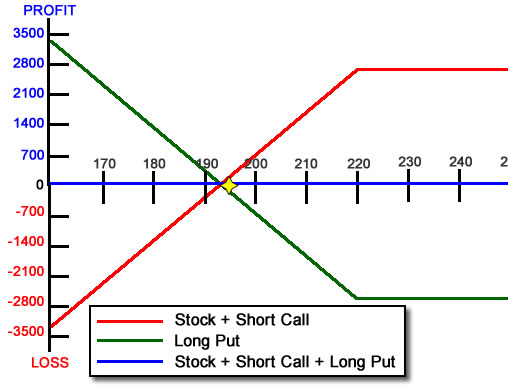Two definitions of the word “synthetic” are:

• produced artificially
• devised, arranged, or fabricated for special situations to imitate or replace usual realities

These definitions can describe “Synthetic Positions” in option trading. To understand synthetic option positions (or “synthetics”), we have to understand the basic relationship between puts and calls:

K + C = U + P + I – D

Where
K = Strike Price
C = Call
U = Underlying stock price
P = Put
I = Interest
D = Dividends

For most situations, we can assume interest and dividends are small enough to ignore. We will simplify our equation by excluding them. There are situations where interest and dividends do affect this equation such as high interest rates, long time periods or large dividends for example.

Simplifying our equation, we now have

K + C = U + P

Since the strike price is arbitrary and a constant, let’s remove it from the equation to see what the relationship is between the Call, Put and Underlying security price boils down to:

C = U + P

Long is positive and short is negative. So we have

• +C = Long Call
• -C = Short Call
• +P = Long Put
• -P = Short Put
• +U = Long Stock
• -U = Short StockThis simple equation let’s you derive the six synthetic relationships quite quickly.

Think back to your algebra class and solve what synthetic you need by putting that variable alone on one side and moving the rest to the other side of the equation. Change the sign when moving from one side to the other of the equation (“jumping the fence as my 8th grade teach used to say”).

We can now show the six basic relationships, solved using basic algebra. They are:

1. Long Call = Long Stock + Long Put     (C = U + P)
2. Short Call = Short Stock + Short Put     (-C = -U – P)
3. Long Put = Long Call + Short Stock     (P = C – U)
4. Short Put = Short Call + Long Stock     (-P = -C + U)
5. Long Stock = Long Call + Short Put     (U = C – P)
6. Short Stock = Short Call + Long Put     (-U = -C + P)

How can we use this knowledge?

Hedge an existing position.

Suppose you have a covered write on a stock but earnings are coming out and you’re worried the stock price might jump around a lot. Or you are going on vacation and don’t want to worry what the market is doing while you’re gone.

Since you know a covered write is identical to a short put synthetically, if you buy a long put, that should completely offset your covered write and completely hedge you while you go through earnings. No need to sell your stock and buy in your short call. Just buy a put. Here’s how that would look:Long stock + Short Call vs Long Put vs Combination

Notice how the blue line is completely flat. That’s the combined position of the long stock, short call and long put.

Reverse Market Directional Bias quickly

Suppose you are bullish on the market and have a Long Call. You change your mind and decide you should be bearish. If you sold your long call and bought long puts, you would have two commissions and two sets of slippage to deal with. Since we know a Long Put = Long Call + Short Stock, all we have to do is SHORT THE STOCK. This changes your position to a synthetic Long Put with one transaction.

This technique is also VERY useful in very fast market conditions. Option bid/ask spreads can really widen during fast markets so your fills closing your Long Call and buying your Long Put might really put you in a hole starting out. Since stocks are very liquid and have very tight bid/ask spreads normally, shorting the stock is a very useful tool to convert your Long Call position during a fast market.

Closing a position with illiquid options using a boxThis happened to me trading 30 Year Treasury Bond futures options (ZB contract) a few years ago. The bid/ask spread of the in-the-money options was really crazy. (Bid \$4, Ask \$6 for example). I had a profit in a position that had started out as a butterfly. I could close most of the position with good fills; however, I had some ITM options with very unfavorable bid/ask spreads. What did I do? I built a box!

I had these options I needed to close:

• A long Put at 118 Strike
• A long Call at the 113 strike

To create a riskless position, I did the opposite:

• Short Call at the 118 Strike
• Short Put at the 113 Strike

I ended up with:

• A long Put + Short Call at the 118 strike = a synthetic Short Stock position
• A Long Call + Short Put at the 113 strike = a synthetic Long Stock position
• Which means I was synthetically Long AND Short the stock (which means there’s no risk left)

I hope you’ve seen how useful synthetic option positions can be. There’s more variations but as long as you understand the basic formula of

Long Call = Long Stock + Long Put

You can re-arrange the equation like in algebra class to put the one variable you need by itself and everything else on the other side of the equation to see what the synthetic is.